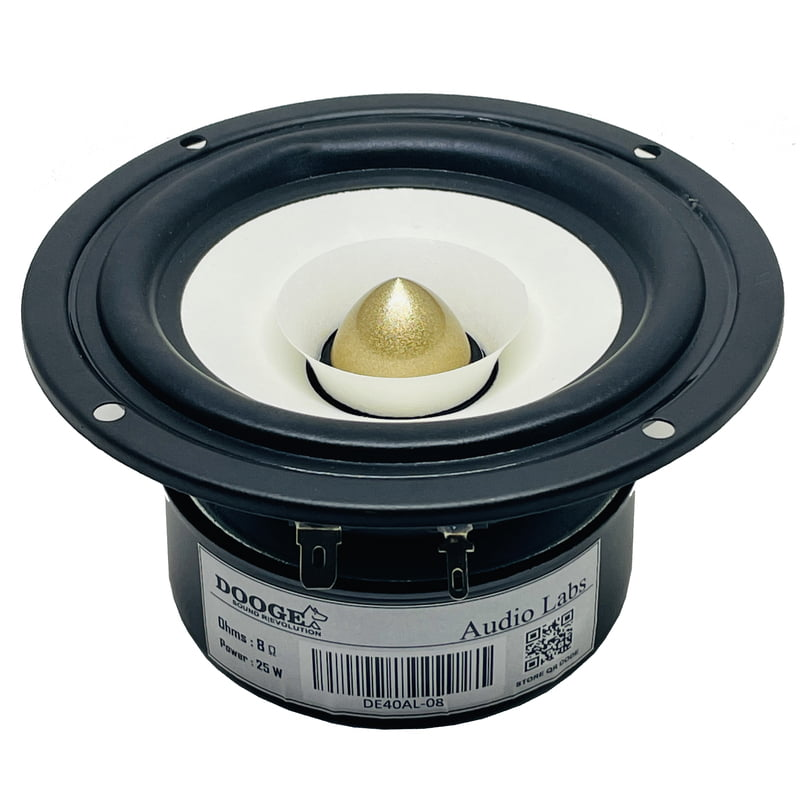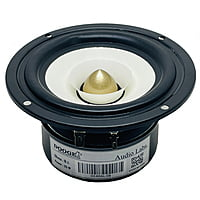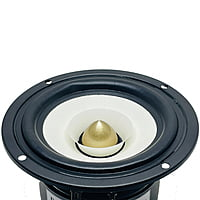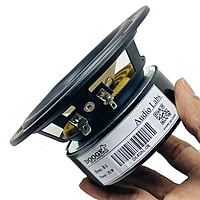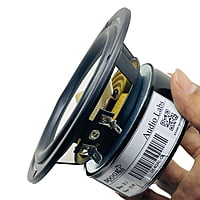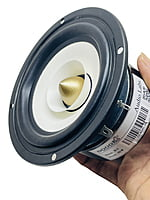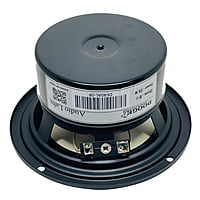# DE40AL-08 Woofer 4" 8Ohms 25 Watts (1 Pcs)

Rs.1,099.00 Rs.1,999.00
Out of stock
4 inch full range speaker coaxial horn unit aluminum bullet 2 layer paper cone home theater satellite

Impedance:8Ohm

Rated Power:25W

Power range:20-30W

frequency response:61Hz-18.5KHz

sensitivity:88dB

f(s) = 122 Hz

Q(ts) = 2.877

V(as) = 1.657 liters (0.05852 cubic feet)

SPL = 78.02 dB SPL 1W/1m

R(e) = 7.368 Ohms

Q(es) = 7.351

SPL = 78.37 dB SPL 2.83 Vrms

C(ms) = 0.388 mm/N

L(e) = 0.1815 mH at 10kHz

Q(ms) = 4.728

BL = 1.837

n(0) = 0.03906 %

M(ms) = 4.39 grams
Product Details
Recommended for 5.1 system making